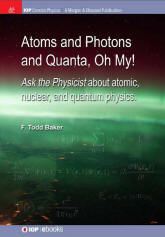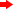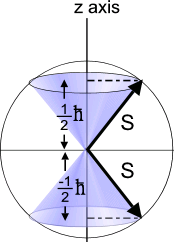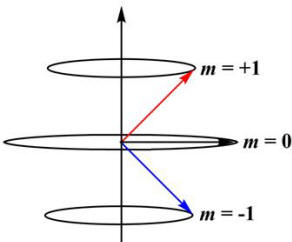# Quantum Physics

With the recent publication of PHYSICS IS... there are now three Ask the Physicist books! Click on the book images below for information on the content of the books and for information on ordering.QUESTION:
in classical physics angular momentum is a variable factor, but why in quantum mechanics angular momenta are quantized. and how its related to spin. I have read that Paul Dirac showed, how changing some relativistic factors in Shroedinger's equation can spontaneously lead to the "spin" concept. but what the spin actually tells us and how we can visualize it.ANSWER:
The reason why orbital angular momentum is quantized is that, when you solve the Schrödinger equation for the atomic wave function, the wave function is not normalizable unless the angular momentum is ħ
√[L(L+1)] where L is an integer. (For our purposes here, "not normalizable" means that the wave function becomes infinite somewhere.) It also turns out that  L is called the orbital angular momentum quantum number and its being an integer has nothing to do with spin except that spin is also an angular momentum. To visualize spin, read two earlier answer (#1 and #2). In relativistic quantum mechanics, the Dirac equation replaces the Schrödinger equation. When you write the Dirac equation for an electron, it turns out that spin is indeed predicted to have an angular momentum quantum number of ½ as is observed experimentally.QUESTION:
Whether light is a particle or wave? Which is right and why?ANSWER:
Light is not a particle or a wave, it is a particle and a wave. This is called wave-particle duality. If you design an experiment to observe a particle, you will observe a particle; and, if you design an experiment to observe a wave, you will observe a wave.QUESTION:
Hi! I am new to Quantum Mechanics and I am a little confuse about the representation of the spin. So if I have a 1/2 spin particle I usually put spin up in the positive direction of Z axis and spin down in the negative one and the probability of getting spin up or down is proportional to the cos^2 of half of the angle between spin and the certain direction. But if I have multiple options for the spin (e.g. in spin one i have spin 1,0,-1) how do I visualize them? I mean If i put upward and downward in the z axis, and at z=0 for spin of 0 I don't get the probability one if I apply the above formula.ANSWER:
I do not know what you mean about the "
cos2 of half of the angle…", but you seem to not know what, e.g., spin ½ means. When you say spin s, this means that the spin angular momentum quantum number is s; this means that the magnitude of that spin angular momentum is S=ħ√[s(s+1)] where ħ is the rationalized Planck constant. But, there is another quantum number, ms=-s, -s+1, -s+2, …, s-1, s which is the quantum number for the z-component of S, Sz=msħ. Hence, the angle θ which the vector makes with the positive z-axis is θ=cos-1(Sz/S). For s=½, θ=cos-1(½/√(3/4))=54.70 and θ=cos-1(-½/√(3/4))=125.30; similarly, for s=1, θ=450, 900, 1350.QUESTION:
Why do wavefunctions need to be normalized?ANSWER:
So that the absolute square of the wave function can be interpreted as a probability density. The integral of
ψ*ψ over all space is then the probability of finding the particle somewhere which, of course, must be 1.QUESTION:
Good afternoon. I started learning quantum mechanics and I have a question about Heinsenberg's principle. I was thinking about a photon created by an electron-pozitron annihilation. As I understand (and I hope I am not wrong here) all observers, no matter their state, can specify the point (and all will specify the same point) where the photon appears, and I think in a Wilson chamber we can do this thing. Anyway, we can specify its position with an error less than infinity. But at the same time we know it's speed with absolute precision, as, assuming we measure it in vacuum, it's speed is invariably c. So the product between (delta)x and (delta)p will be 0, as (delta)x is not infinity and (delta)p is 0. In other words we can predict it's position with a certain accuracy knowing at the same time it's speed (I am not sure, but i think that the (delta)p is not a vector but a scalar quantity). What is wrong about this as it seems the Heinsenbrg's principle is violated?ANSWER:
The mass of a photon is zero but it has momentum. Therefore your notion that linear momentum p is mass m times velocity v must be wrong; the relativistically correct expression for momentum is p=mv/ √[1-(v/c)2] where c is the speed of light. For a photon, this is a little tricky because p=0/0, but you can also write that E2=p2c2+(mc2)2 where E is the energy of the a particle of mass m, so p=E/c if m=0; since E=hf for a photon, where h is Planck's constant and f is the frequency, the linear momentum of a photon is p=hf/c . Therefore the frequency of the photon must be uncertain according to the uncertainty principle. Since the energy of a photon is hf, there will be an uncertainty in the energy of the photon.